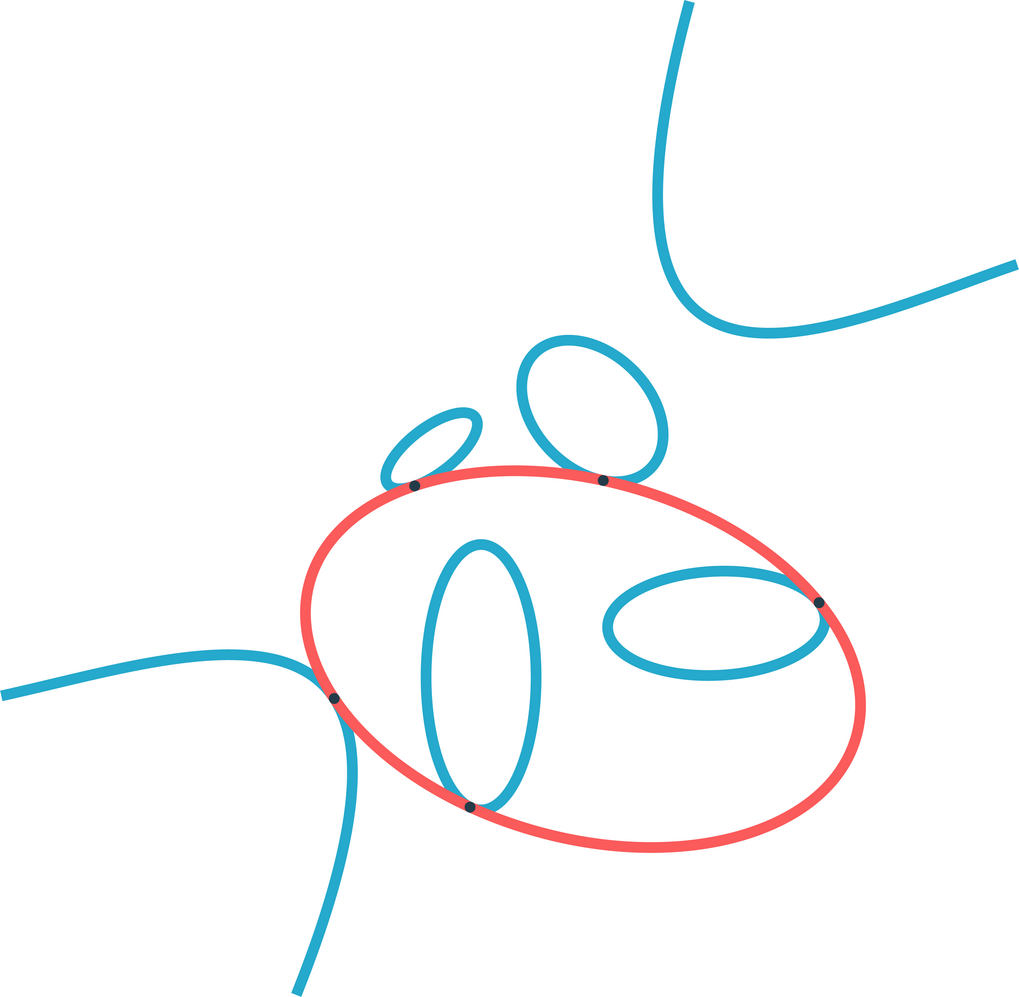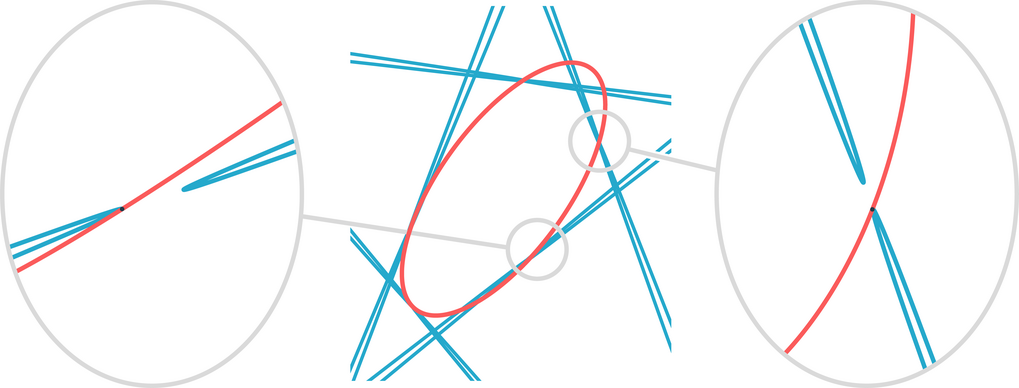# Blurred lines – mathematical software unites theory and practice

Numerical solutions for systems of polynomial equations and answers to questions of theoretical mathematics

January 14, 2020

Classically there is a clear distinction between theoretical and applied mathematics in the classification of different fields in the mathematical sciences. Bernd Sturmfels at the Max Planck Institute for Mathematics in the Sciences, along with Paul Breiding and Sascha Timme at the Technical University of Berlin present a novel approach that illustrates how this line can be blurred. They developed a custom software, which is not only able to compute numerical solutions for systems of polynomial equations, but can also be employed to answer classical questions of theoretical mathematics. Their results made the cover story of the January issue of the "Notices of the American Mathematical Society".

The article "3264 Conics in a Second" exemplifies how mathematical software can serve as a bridge between theoretical problems and applied methods. Besides creating the extensive numerical software package "HomotopyContinuation.jl", the authors also established an easily accessible website, which vividly showcases the various applications of the software. One of these applications, in turn, constitutes the solution to a classical geometric problem, which was made even more accessible with a webapp.

## Steiner's conic problem

The published paper revolves around a classical geometric problem from the perspective of modern numerical algorithms. In 1848 the mathematician Jakob Steiner posed the question of finding the number of conics tangent to five given conics. A conic is a planar curve given by the intersection of the surface of a cone with a plane, yet it also constitutes the zero set of a quadratic equation in two variables. Even though Steiner's question initially seems quite academically rigorous in nature it is pertinent to modern applications. The conic problem is regarded as the origin of modern intersection theory. This theory then forms the foundation for modern algorithms to compute the roots of polynomial systems, which is a fundamental problem in many applied fields: robotics, material sciences, machine learning, biology or dynamical systems theory are just a few exemplary fields, where polynomial equations need to be solved.

## Software package HomotopyContinuation.jlThe figure illustrates a red ellipse that is tangent to four blue ellipses and one blue hyperbola. © Thomas Endler, MPI for Mathematics in the Sciences

Exactly these numerical computations form the basis of the authors specialized software package HomotopyContinuation.jl, which was developed for Julia, a dynamic programming language focused on high-performance numerical analysis. The accompanying website not only offers an all-encompassing software manual, but also contextualizes the software through an assortment of applications ranging from computer vision, robotics, chemistry, mathematics of data and algebraic geometry. A variety of guides introduce the user to the software features and explain how they can be applied to numerous problems. Such as Steiner's conic problem, which can be formulated as a problem of finding the roots of a polynomial system. The customized software package is able to compute these solutions within a mere second.

The scientists at the Max Planck Institute and the Technical University of Berlin created a web interface accompanying their publication which allows the reader to easily compute the 3264 solutions for their chosen conics (see juliahomotopycontinuation.org/diy). This innovative form of science communication is a novelty for publications in the Notices. The authors were able to use their software to compute exact equations for arrangements with real solutions to Steiner's conic problem. Thus, they strikingly demonstrated how theoretical results involve numerical procedures, as well as the possible application of numerical methods in proofs of specific theoretical results.The figure shows the five blue conics (they are solely thin hyperbolas) tangent to one of the 3264 real conics in red. © Thomas Endler, MPI for Mathematics in the Sciences
Go to Editor View